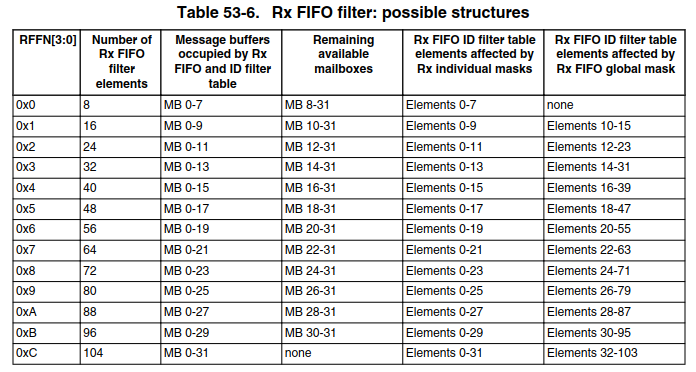# S32K CAN Tx issue

cancel
Showing results for
Did you mean:

## S32K CAN Tx issue

443 ViewsContributor III

Hello，

I used CAN FIFO  interrupt mode for reception. Set the MBs is 32.  MCU model is S32K148.

It has the following conditions：

1. When I set the RxFIFO ID filters numbers is 8. The Tx can use the MailBox is from number 8 to number 31.

2. When I set the RxFIFO ID filters numbers is 16. The Tx can use the MailBox is from number 10 to number 31.

3. When I set the RxFIFO ID filters numbers is 24. The Tx can use the MailBox is from number 12 to number 31.

4. When I set the RxFIFO ID filters numbers is 32. The Tx can use the MailBox is from number 14 to number 31.

5. When I set the RxFIFO ID filters numbers is 40. The Tx can use the MailBox is from number 16 to number 31.

5. When I set the RxFIFO ID filters numbers is 48. The Tx can use the MailBox is from number 18 to number 31.

5. When I set the RxFIFO ID filters numbers is 56. The Tx can use the MailBox is from number 20 to number 31.

5. When I set the RxFIFO ID filters numbers is 64. The Tx can use the MailBox is from number 22 to number 31.

5. When I set the RxFIFO ID filters numbers is 72. The Tx can use the MailBox is from number 24 to number 31.

5. When I set the RxFIFO ID filters numbers is 80. The Tx can use the MailBox is from number 26 to number 31.

5. When I set the RxFIFO ID filters numbers is 88. The Tx can use the MailBox is from number 28 to number 31.

5. When I set the RxFIFO ID filters numbers is 96. The Tx can use the MailBox is from number 30 to number 31.

5. When I set the RxFIFO ID filters numbers is 104. The Tx can't send successfully.

I don't know how the Tx mailbox has anything to do with the number of RXFIFO filters?

Where is the description?

Thanks！

244 ViewsNXP TechSupport

Hi,

each MB can be set either for RX or TX operation. If the RXFIFO is enabled too, more MBs are occupied by RXFIFO functionality (RXFIFO and ID filter table) depending the CTRL2[RFFN] setting. Thus less MBs left for RX or TX operation, as Table 53-6 states.BR,

Petr